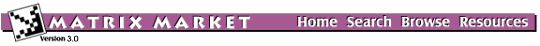## XLATMR: Random Matrices from the LAPACK Test Suite

### from the Independent Sets and Generators

Matrix Generator XLATMR
Source: LAPACK
Discipline: Numerical linear algebra
Language: Fortran
Output format: Matrix Market format, column major dense, column major dense packed

DLATMR/ZLATMR generates random matrices of various types, and was developed to aid in testing the dense/structured sparse numerical algorithms in LAPACK. These routines are distributed as part of the LAPACK Test Suite, which is bundled with the complete library distribution, and, for convenience, are available here; see Links.

Interactive generation of DLATMR/ZLATMR matrices in Matrix Market format (with size restriction) and sample driving programs is available through the CGI Interface to DLATMR/ZLATMR.

Random dense/sparse matrices are generated in DLATMR/ZLATMR by applying the following sequence of operations:

1. Generate a matrix A with random entries of distribution DIST which is symmetric if SYM='S', Hermitian if SYM='H', and nonsymmetric if SYM='N'.
2. Set the diagonal to D, where D may be input or computed according to MODE, COND, DMAX and RSIGN as described below.
3. Grade the matrix, if desired, from the left and/or right as specified by GRADE. The inputs DL, MODEL, CONDL, DR, MODER and CONDR also determine the grading as described below.
4. Permute, if desired, the rows and/or columns as specified by PIVTNG and IPIVOT.
5. Set random entries to zero, if desired, to get a random sparse matrix as specified by SPARSE.
6. Make A a band matrix, if desired, by zeroing out the matrix outside a band of lower bandwidth KL and upper bandwidth KU.
7. Scale A, if desired, to have maximum entry ANORM.
8. Pack the matrix if desired. Options specified by PACK are:
• no packing
• store the lower half columnwise (if symmetric or Hermitian)

Parameters:

 M number of rows N number of columns DIST distribution of random values SYM symmetry D diagonal entries MODE diagonal selection control COND diagonal selection parameter DMAX diagonal scaling parameter RSIGN application of random sign GRADE grade control DL left grading matrix entries MODEL left grading matrix selection CONDL left grading matrix selection parameter DR right grading matrix entries MODER right grading matrix selection CONDR right grading matrix selection parameter PIVTNG pivoting selection control IPIVOT pivoting selection parameter SPARSE degree of sparsity KL lower bandwidth KU upper bandwidth ANORM matrix scaling factor PACK output format